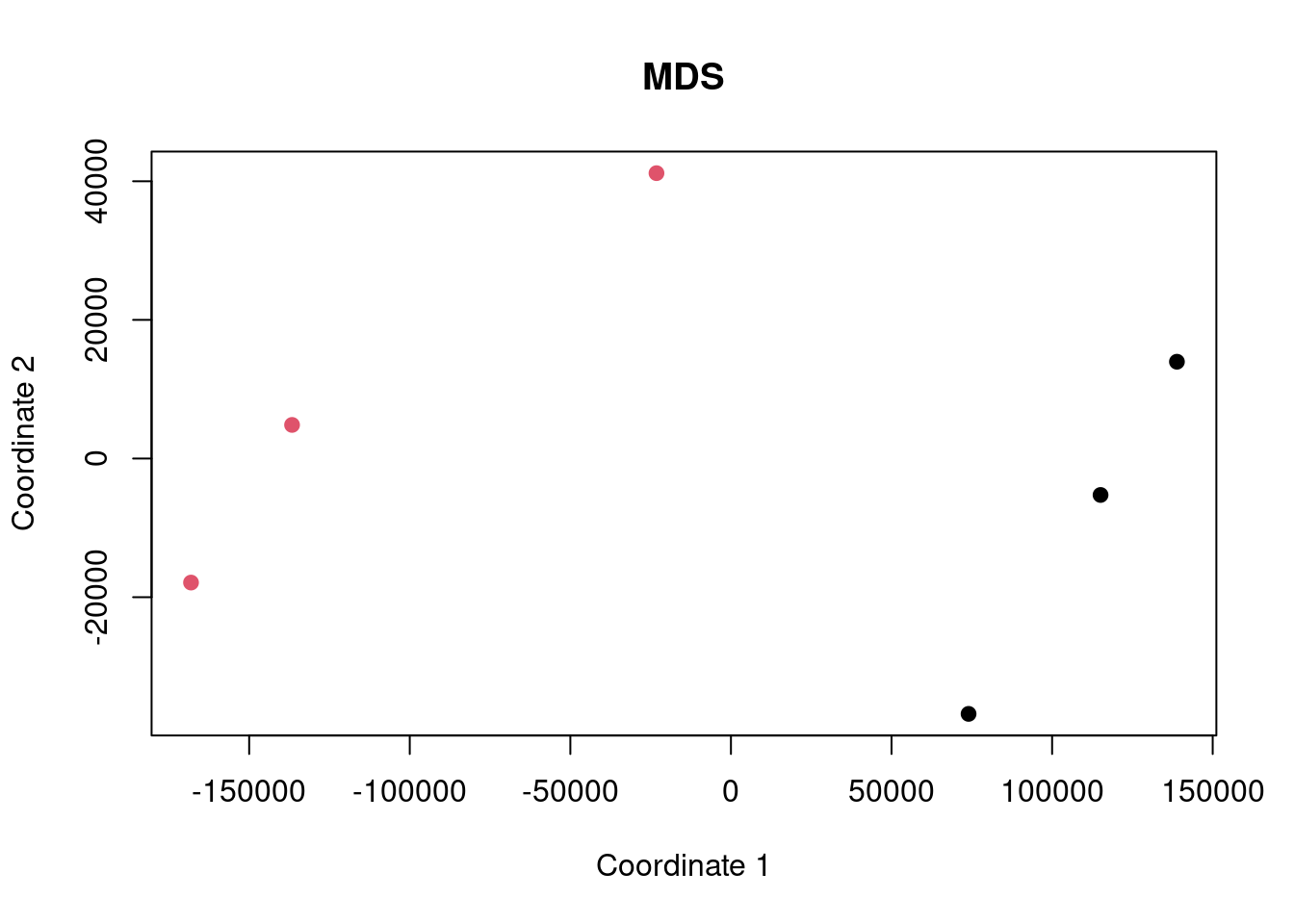## Intro

Here we are performing an analysis of some gene expression data to demonstrate the difference between ORA and FCS methods and to highlight the differences caused by improper background gene set use.

The dataset being used is SRP038101 and we are comparing the cells expressing a set7kd shRNA construct (case) compared to the scrambled construct (control).

Data are obtained from http://dee2.io/

``````suppressPackageStartupMessages({
library("getDEE2")
library("DESeq2")
library("clusterProfiler")
library("mitch")
library("kableExtra")
library("eulerr")
})``````

## Get expression data

I’m using some RNA-seq data looking at the effect of Set7 knockdown on HMEC cells.

``````name="SRP096177"
samplesheet <- mdat[grep("SRP096177",mdat\$SRP_accession),]
samplesheet<-samplesheet[order(samplesheet\$SRR_accession),]
samplesheet\$trt<-as.factor(c(1,1,1,0,0,0))
s1 <- samplesheet

s1 %>% kbl(caption = "sample sheet") %>% kable_paper("hover", full_width = F)``````
sample sheet
SRR_accession QC_summary SRX_accession SRS_accession SRP_accession Sample_name GEO_series Library_name trt
379112 SRR5150592 PASS SRX2468682 SRS1901000 SRP096177 GSM2448982 GSE93236 1
379113 SRR5150593 PASS SRX2468683 SRS1901005 SRP096177 GSM2448983 GSE93236 1
379114 SRR5150594 PASS SRX2468684 SRS1901001 SRP096177 GSM2448984 GSE93236 1
379115 SRR5150595 PASS SRX2468685 SRS1901002 SRP096177 GSM2448985 GSE93236 0
379116 SRR5150596 PASS SRX2468686 SRS1901003 SRP096177 GSM2448986 GSE93236 0
379117 SRR5150597 PASS SRX2468687 SRS1901004 SRP096177 GSM2448987 GSE93236 0
``w<-getDEE2("hsapiens",samplesheet\$SRR_accession,metadata=mdat,legacy = TRUE)``
``````## For more information about DEE2 QC metrics, visit
##     https://github.com/markziemann/dee2/blob/master/qc/qc_metrics.md``````
``````x<-Tx2Gene(w)
x<-x\$Tx2Gene

# save the genetable for later
gt<-w\$GeneInfo[,1,drop=FALSE]
gt\$accession<-rownames(gt)

# counts
x1<-x[,which(colnames(x) %in% samplesheet\$SRR_accession)]``````

Here show the number of genes in the annotation set, and those detected above the detection threshold.

``````# filter out lowly expressed genes
x1<-x1[which(rowSums(x1)/ncol(x1)>=(10)),]
nrow(x)``````
``##  39297``
``nrow(x1)``
``##  15607``

Now multidimensional scaling (MDS) plot to show the correlation between the datasets. If the control and case datasets are clustered separately, then it is likely that there will be many differentially expressed genes with FDR<0.05.

``plot(cmdscale(dist(t(x1))), xlab="Coordinate 1", ylab="Coordinate 2", pch=19, col=s1\$trt, main="MDS")``## Differential expression

Now run DESeq2 for control vs case.

``y <- DESeqDataSetFromMatrix(countData = round(x1), colData = s1, design = ~ trt)``
``## converting counts to integer mode``
``y <- DESeq(y)``
``## estimating size factors``
``## estimating dispersions``
``## gene-wise dispersion estimates``
``## mean-dispersion relationship``
``## final dispersion estimates``
``## fitting model and testing``
``````de <- results(y)
de<-as.data.frame(de[order(de\$pvalue),])
rownames(de)<-sapply(strsplit(rownames(de),"\\."),"[[",1)
head(de) %>% kbl() %>% kable_paper("hover", full_width = F)``````
baseMean log2FoldChange lfcSE stat pvalue padj
ENSG00000168542 1259.1001 2.7325696 0.0952126 28.69967 0 0
ENSG00000164692 11379.8760 2.2961488 0.0887385 25.87546 0 0
ENSG00000172531 2130.9201 -1.7175061 0.0711786 -24.12951 0 0
ENSG00000106484 4549.7360 1.2253275 0.0581383 21.07606 0 0
ENSG00000130508 10277.9876 -0.9442904 0.0530537 -17.79877 0 0
ENSG00000163017 300.4833 4.8785546 0.2742716 17.78731 0 0

Now let’s have a look at some of the charts showing differential expression. In particular, an MA plot and volcano plot.

``````maplot <- function(de,contrast_name) {
sig <-subset(de, padj < 0.05 )
up <-rownames(subset(de, padj < 0.05 & log2FoldChange > 0))
dn <-rownames(subset(de, padj < 0.05 & log2FoldChange < 0))
GENESUP <- length(up)
GENESDN <- length(dn)
DET=nrow(de)
SUBHEADER = paste(GENESUP, "up, ", GENESDN, "down", DET, "detected")
ns <-subset(de, padj > 0.05 )
plot(log2(de\$baseMean),de\$log2FoldChange,
xlab="log2 basemean", ylab="log2 foldchange",
pch=19, cex=0.5, col="dark gray",
main=contrast_name, cex.main=0.7)
points(log2(sig\$baseMean),sig\$log2FoldChange,
pch=19, cex=0.5, col="red")
}

make_volcano <- function(de,name) {